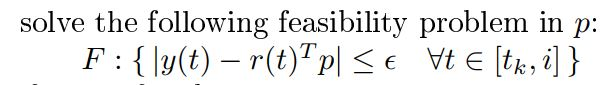# How to write many inequality constraints in a matrix form?

i have the following inequality constraints , instead of using a loop , i want to make it in matrix form , how to do that so that both solutions will be samey is a scalar , r and p are vectors

I think if you use a vector `y`, matrix `r`, producing a vector `y-r'*p`, and apply `norms` to it, that ought to do what you want. I leave the details to you.

applying norms or the abs operator ??

You can apply `norms` so that the result will be a vector of two-norms, with each two-norm being applied in the dimension which has only a single element, which will therefore be the same as `abs`.

For instance

``````cvx_begin
variable x(3)
norms(x,[],2)
``````

ans =

``````cvx convex expression (3x1 vector)
``````

In your case, apply this to the column vector `y - r'*p`, in place of my `x` above, where y is a column vector and `r` is a suitable matrix.

So
`norms(y - r'*p,[],2) <= epsilon`

the right hand side should be the one vector times eps i think , coz we have multiconstraints , and what will the norm give on the left hand side

Assume there are n constraints.
`y` is n by 1. `p` is m by 1. `r` is m by n (i.e., an m by 1 column for each constraint).
So `y - e'*p` is an n by 1 vector.
`norms(y - r'*p,[],2)` is an n by 1 vector, each element of which is the absolute value of the corresponding element of `y - r'*p`.

The use of `ones(n,1) on the RHS is optional, and is automatically applied in MATLAB and CVX. I omitted it to reduce clutter in the program.

1 Like

Actually, `abs` can be used directly as a more straightforward alternative to `norms`, and will be applied per element, thereby producing a vector output from a vector input.

`abs(y - r'*p) <= epsilon`

1 Like